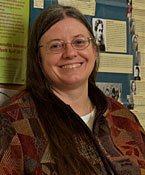myMCLA LOGON
DIRECTORY | SUPPORT MCLADr. Fredricka Bennett

Professor, Mathematics

Research/ Creative Interests

Graph Theory, History of Mathematics, Mathematical Statistics, Mathematics Education, Mathematical Modeling of the Environment, and Financial Mathematics

Contact Information

F.Bennett@mcla.edu

Office: Bowman Hall 105B

Education

University of Massachusetts, Amherst, MA
Ph.D in Mathematics (Thesis Advisor: Dr. Walter Rosenkrantz) September 1983. Thesis: On a Sequence of Markov Processes Converging to a Multivariate Ornstein-Uhlenbeck Process.

University of Massachusetts, Amherst, MA
M.A in Mathematics February 1982.

B.A. in Mathematics and in Economics, ay 1976.

Courses Taught

Concepts of College Mathematics

Mathematics for the Liberal Arts

Calculus I

Introduction to Statistics

Set Theory and Mathematical Logic

Linear Algebra

Linear Programming

How to Teach Mathematics

Calculus II, Calculus III

Probability Theory

Statistical Analysis

Graph Theory

Geometry

Game Theory

Number Theory

Algebraic Structures

Linear Algebra II

Computability and Complexity

History of Mathematics

Differential Equations

Calculus IV Topology

Complex Variables Operations Research Real Analysis

Cryptology

Mathematical Modeling

Issues and Trends in Mathematics Education

First Year Seminar - Science and Technology Problem Solving and Numeration

Data Analysis.